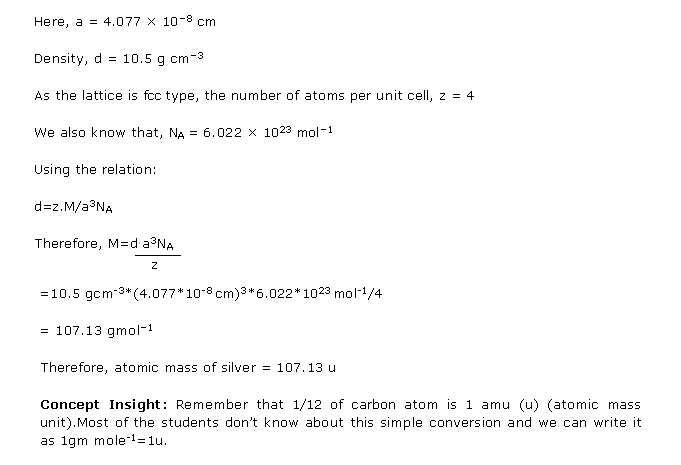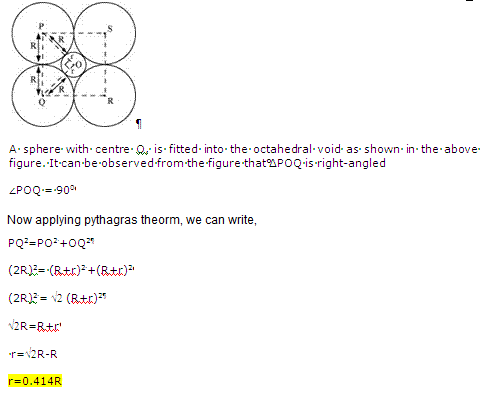# NCERT Solutions for Class 12-science Chemistry Chapter 1 - The Solid State

Page / Exercise

## Chapter 1 - The Solid State Exercise 4

Solution 1Solution 2Solution 3Solution 4 OldSolution 4## Chapter 1 - The Solid State Exercise 7

Solution 6Solution 7Solution 8Solution 9## Chapter 1 - The Solid State Exercise 14

Solution 10Solution 11Solution 12Solution 13## Chapter 1 - The Solid State Exercise 23

Solution 14## Chapter 1 - The Solid State Exercise 24

Solution 15Solution 16Solution 17## Chapter 1 - The Solid State Exercise 31

Solution 18

31Solution 19Solution 20Solution 21Solution 22Solution 23## Chapter 1 - The Solid State Exercise 32

Solution 1Solution 2Solution 3Solution 4Solution 5Solution 6## Chapter 1 - The Solid State Exercise 33

Solution 7Solution 8Solution 9Solution 10Solution 11Solution 12Solution 13Solution 14Solution 15Solution 16Solution 17Solution 18Solution 19Solution 20## Chapter 1 - The Solid State Exercise 34

Solution 21Solution 22Solution 23Solution 24Solution 25Solution 26